• 2021-04-21 23:05:37

arma()

功能：估计ARMA时间序列模型参数

格式：

m = armax(data, orders);

m = armax(data, 'na', na, 'nb', nb, 'nc', nc, 'nk', nk)

m = armax(data, orders, 'Property1', Value1,..., 'PropertyN', ValueN)

说明

模型描述为 A(q)y(t)=B(q)u(t-nk)+C(q)e(t)

data:输入／输出时间序列

orders:ARMA模型阶数结构，形式为orders = [na nb nc nk]

其中na,nb,nc为模型参数，nk为延迟。

property:模型估计时的参数设置

xcorr()和autocorr()

摘自博客

例如：A=[1,2,3,4] xcorr(A)=[4,10,20,30,20,10,4]

注：xcorr.length=A.lenth*2-1 ，且为对称

上面的矩阵，最后得到7个结果，其中第4个值最大11+22+33+44 = 30 。而第三个和第五个分别是间隔正负1的结果也就是12+23+34 = 20，21+32+43 = 20 。第二个和第六个分别是间隔正负2也就是13+24=11，31+42 = 11。第一个和第七个分别是间隔正负3也就是14 = 4 ，41=4

autocorr(Series,nLags,M,nSTDs) //计算并绘制时间序列的自相关函数

Series为时间序列

nLags--延迟，当nLags=[]或缺省时，计算ACF时在延迟点0、1、2、。。。、T处，T=min([20 length(Series-1)])

M--非负整数，表示在多大延迟时理论ACF为0.autocorr假设序列为MA(M)，并且使用Bartlett估计方法来计算大于M的延迟的标准误差。如果M=[]或缺省，则为0，函数假设序列为高斯白噪声。

nSTDs--样本ACF估计误差的标准差。

ACF--样本自相关函数

Lags--与ACF(0,1,2，。。。，nLags)相对应的延迟

Bounds--置信区间的近似上下限，假设序列为MA(M)过程。

autocorr是对序列减去均值后做的自相关，最后又进行了归一化。而且由于自相关本身是偶函数，autocorr只是取了以中点n为起始的后面n个序列。

更多相关内容
• ARIMA模型MATLAB实现代码，通过一批现有数据（本代码中batch=100），向后预测后n个数据，n越大，预测效果越差。代码可以直接运行，有实例支持，可以很快上手。压缩包包括以下三部分：主程序（ARIMA_main.m），辅助...
• ARIMA模型-matlab代码，可以根据自己的实际情况进行参数调节，实现所需要的效果。 ARIMA模型-matlab代码，可以根据自己的实际情况进行参数调节，实现所需要的效果。
• arimamatlab代码使用 ARIMA-LSTM 混合模型进行相关预测 我们应用了 ARIMA-LSTM 混合模型来预测两种资产的未来价格相关系数 我的论文草稿上传到 . 我愿意对我的工作发表任何评论。 请给我发电子邮件。 我真的很感激...
• ## ARIMA模型MATLAB代码

热门讨论 2012-12-06 18:58:29
• ARIMA：自回归平均移动模型MATLAB代码函数实现，可以直接输入参数进行运行。希望能给你带来一些帮助，但我最近下载资源积分不够，收取一些积分希望谅解，代码出什么问题，告诉我，我帮你调。
• 时间序列预测建模，移动平滑、指数平滑、等模型的描述讲解和matlab程序实现代码arima、arma等等
• 此压缩包中包括2018年华为软赛初赛练习数据，数据预处理及应用MATLAB自带的ARIMA函数进行算法可行性验证。
• arimamatlab代码个人家庭电力的时间序列预测 日期集： 数据是在 2006 年 12 月至 2010 年 11 月（47 个月）期间以一分钟采样率收集的。 六个自变量（电量和子计量值）一个数值因变量 全球有功功率有 2,075,259 个...
• 时间序列预测建模，ARIMA模型MATLAB程序实现代码
• ARIMA 预测模型 训练集和预测集 ARIMA模型全称为自回归积分滑动平均模型(Autoregressive Integrated Moving Average Model,简记ARIMA)，是由博克思(Box)和詹金斯(Jenkins)于70年代初提出一著名时间序列（Time-series...
• ar模型matlab代码MATLAB中的11种经典时间序列预测方法 凯文 机器学习实施的兴起，引起了不同行业的兴趣，将其用于时间序列问题的分类和预测。 在探索时间序列的机器学习方法之前，最好确保已尝试使用分类和统计时间...
• 这段是实现预测的关键代码，需要大家根据个人情况编写剩余代码 参数定义 ： x为原始序列数据 y为进行周期差分变换后的x w为差分运算之后的y 话不多说，直接上代码，后面有分段的代码讲解 figure(1); plot...

这段是实现预测的关键代码，需要大家根据个人情况编写剩余代码

参数定义 ： x为原始序列数据

y为进行周期差分变换后的x

w为差分运算之后的y

话不多说，直接上代码，后面有分段的代码讲解

figure(1);
plot(x,'k-h');
% set(gca,'XTicklabel',{'2005/01','2006/08','2008/04','2009/12','2011/08','2013/04', ...
%     '2014/12','2016/08','2018/04','2019/12','2021/08'});
% xlabel('Time');
% ylabel('Elevation');
% legend('Elevation of Lake Mead : 2005 - 2020');
grid on;
title('Raw data image') %原始数据图像
% subplot(1,2,2)
figure(2);
autocorr(x)
title('Autocorrelation function graph') % 自相关函数图像

%做1阶4步差分
s=12;  %周期s=12
n=18;  %预报数据的个数
m1=length(x); % x的个数
for i=s+1:m1
y(i-s)=x(i)-x(i-s);  % 进行周期差分变换
end
w=diff(y,1); % 差分运算，消除趋势性
m2=length(w); w的个数
figure(3);
% subplot(1,2,1)
plot(w);
grid on;
% set(gca,'XTicklabel',{'2005/01','2006/08','2008/04','2009/12','2011/08','2013/04', ...
%     '2014/12','2016/08','2018/04','2019/12','2021/08'});
% xlabel('Time');
% ylabel('Elevation');
% legend('Elevation of Lake Mead Data (2005 - 2020) after The differential');
title('Differential post sequence image') % 差分后序列图像
% subplot(1,2,2)
figure(4);
autocorr(w)
title('Autocorrelation function graph')

% norm test  需要序列服从正态分布
figure(5);
normplot(x);
xlabel('Elevation Data');
ylabel('Posibility');
title('Normal probability graph');
%% select the model

k = 0;
for i = 0 : 3  % 确定模型结构
for j = 0 : 3
if i == 0 & j == 0
continue;
elseif i == 0
ToEstMd = arima('MALags',1 : j,'Constant',0);
elseif j == 0
ToEstMd = arima('ARLags',1 : i,'Constant',0);
else
ToEstMd = arima('ARLags',1 : i,'MALags',1 : j,'Constant',0);
end
k = k + 1;R(k) = i;M(k) = j;
[EstMd,EstParamCov,logL,info] = estimate(ToEstMd,w');
numParams = sum(any(EstParamCov));
[aic(k),bic(k)] = aicbic(logL,numParams,m2);
end
end

fprintf('R,M,AIC,BIC的对应值如下\n%f'); % 根据AIC、BIC准则定阶
check = [R',M',aic',bic']
r = input('输入阶数R = ');m = input('输入阶数M = ');
ToEstMd = arima('ARLags',1 : r,'MALags',1:m,'Constant',0); %指定模型的结构

%% estimate && forecast && results
[EstMd,EstParamCov,logL,info] = estimate(ToEstMd,w');%模型拟合
w_Forecast = forecast(EstMd,n,'Y0',w');
yhat = y(end) + cumsum(w_Forecast); %一阶差分的还原值
for j = 1:n
x(m1 + j) = yhat(j) + x(m1+j-s); %x的预测值
end
xhat = x(m1 + 1 : end); % 提取x预测的值

1.首先是将序列变为平稳的

figure(1);
plot(x,'k-h');
% set(gca,'XTicklabel',{'2005/01','2006/08','2008/04','2009/12','2011/08','2013/04', ...
%     '2014/12','2016/08','2018/04','2019/12','2021/08'});
% xlabel('Time');
% ylabel('Elevation');
% legend('Elevation of Lake Mead : 2005 - 2020');
grid on;
title('Raw data image') %原始数据图像
% subplot(1,2,2)
figure(2);
autocorr(x)
title('Autocorrelation function graph') % 自相关函数图像

%做1阶4步差分
s=12;  %周期s=12
n=18;  %预报数据的个数
m1=length(x); % x的个数
for i=s+1:m1
y(i-s)=x(i)-x(i-s);  % 进行周期差分变换
end
w=diff(y,1); % 差分运算，消除趋势性
m2=length(w); w的个数
figure(3);
% subplot(1,2,1)
plot(w);
grid on;
% set(gca,'XTicklabel',{'2005/01','2006/08','2008/04','2009/12','2011/08','2013/04', ...
%     '2014/12','2016/08','2018/04','2019/12','2021/08'});
% xlabel('Time');
% ylabel('Elevation');
% legend('Elevation of Lake Mead Data (2005 - 2020) after The differential');
title('Differential post sequence image') % 差分后序列图像
% subplot(1,2,2)
figure(4);
autocorr(w)
title('Autocorrelation function graph')


Figure 1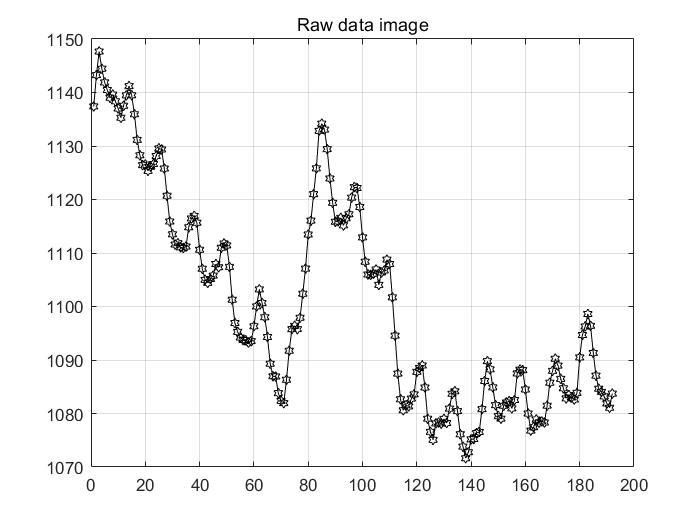可以看到原序列具有显著的趋势，初步判断为非平稳序列。

再看自相关函数图：

Figure 2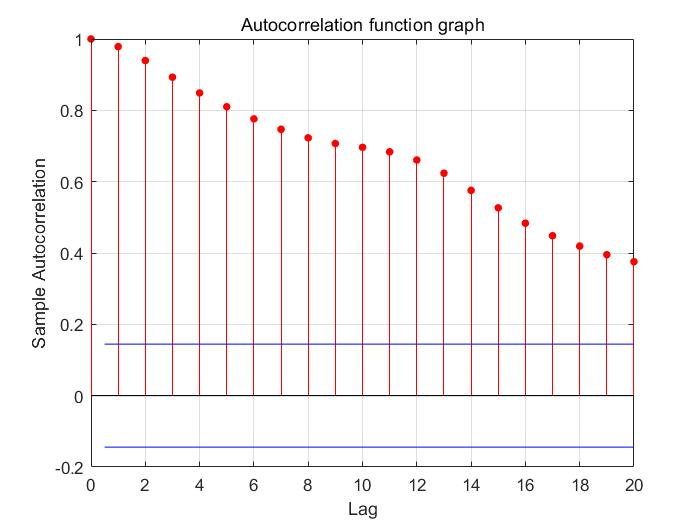可以看到自相关函数图并未较快的衰减为0，因此该序列并非为平稳的。

1.1 确定季节性周期时间，进行周期性差分变换

确定序列为非平稳之后，在处理平稳性之前先需要做一件事：消除周期性、季节性，这里就用到了差分变换

%做1阶4步差分
s=12;  %周期s=12
n=18;  %预报数据的个数
m1=length(x); % x的个数
for i=s+1:m1
y(i-s)=x(i)-x(i-s);  % 进行周期差分变换
end

1.2 如何变为平稳

利用差分运算，对数据进行一阶差分运算，具体用diff函数实现

w=diff(y,1); % 差分运算，消除趋势性
m2=length(w); w的个数
figure(3);
% subplot(1,2,1)
plot(w);
grid on;
% set(gca,'XTicklabel',{'2005/01','2006/08','2008/04','2009/12','2011/08','2013/04', ...
%     '2014/12','2016/08','2018/04','2019/12','2021/08'});
% xlabel('Time');
% ylabel('Elevation');
% legend('Elevation of Lake Mead Data (2005 - 2020) after The differential');
title('Differential post sequence image') % 差分后序列图像
% subplot(1,2,2)
figure(4);
autocorr(w)
title('Autocorrelation function graph')

这里画了两张图，用plot和autocorr函数实现的

Figure 3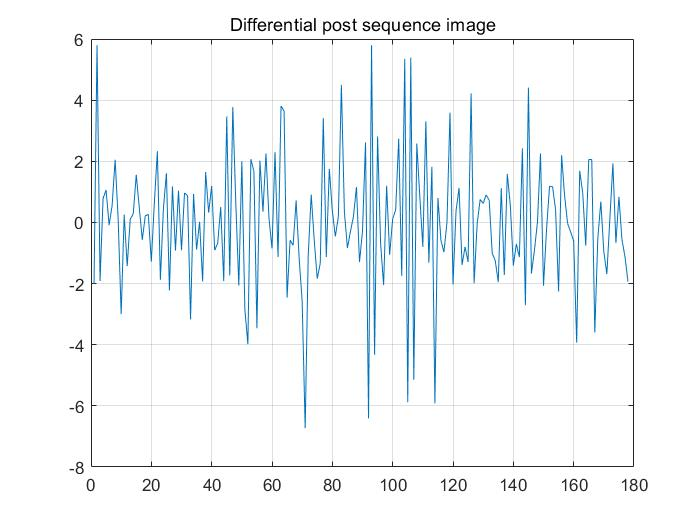Figure 4可以看到一阶差分之后数据在某个区间内波动，且有界，无明显趋势及周期性特征，判断一阶差分之后序列平稳。

2.正态性检验

% norm test  需要序列服从正态分布
figure(5);
normplot(x);
xlabel('Elevation Data');
ylabel('Posibility');
title('Normal probability graph');

Figure 5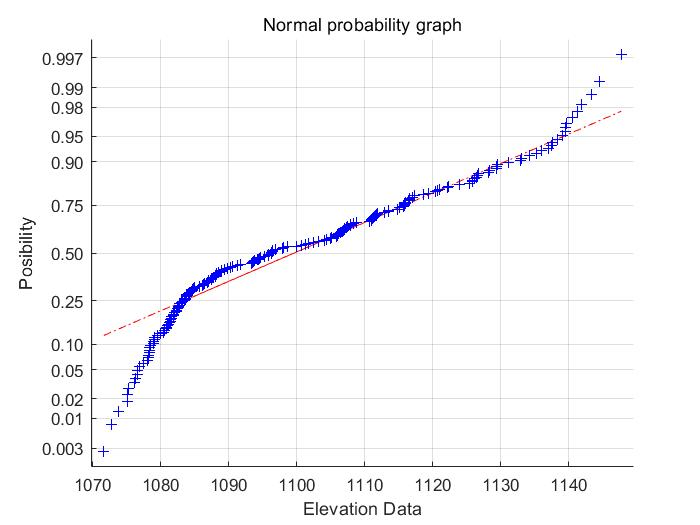可以发现数据基本都位于标线附近，可以认为数据服从正态分布(其实不太严谨但我直接用了hh，差不多就行应该问题不大)

3.模型的遍历选择

%% select the model

k = 0;
for i = 0 : 3  % 确定模型结构
for j = 0 : 3
if i == 0 & j == 0
continue;
elseif i == 0
ToEstMd = arima('MALags',1 : j,'Constant',0);
elseif j == 0
ToEstMd = arima('ARLags',1 : i,'Constant',0);
else
ToEstMd = arima('ARLags',1 : i,'MALags',1 : j,'Constant',0);
end
k = k + 1;R(k) = i;M(k) = j;
[EstMd,EstParamCov,logL,info] = estimate(ToEstMd,w');
numParams = sum(any(EstParamCov));
[aic(k),bic(k)] = aicbic(logL,numParams,m2);
end
end


当i == 0，j == 0的时候跳过，其他值的时候进入，(0,1)(0,2)(0,3)(1,0)(1,1)(1,2)......等15(16 - 1)个挨个参数带入arima函数进行计算，同时计算出AIC和BIC值，保存下来。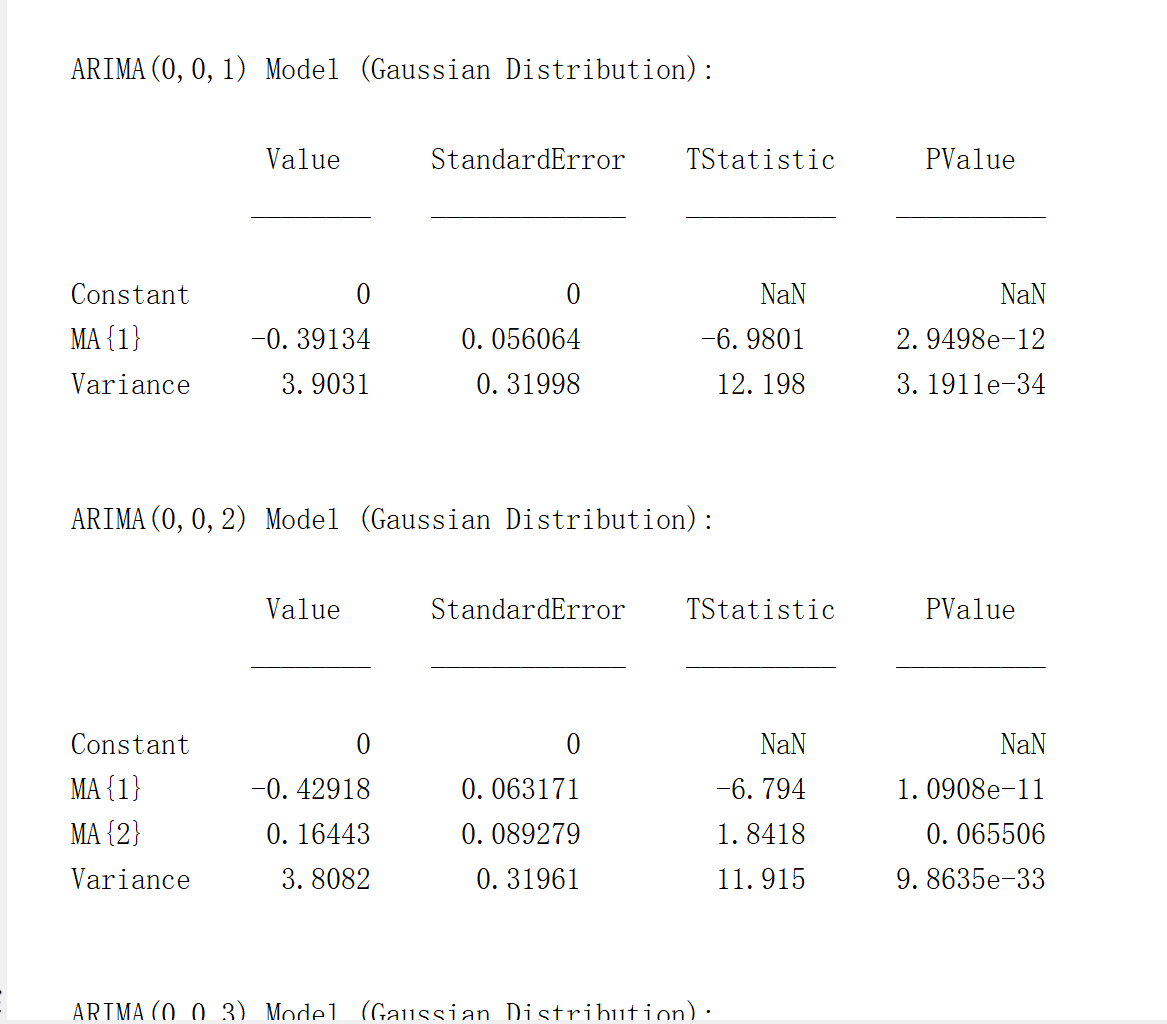--------------------------------------------------------------------------------------------------------------------------------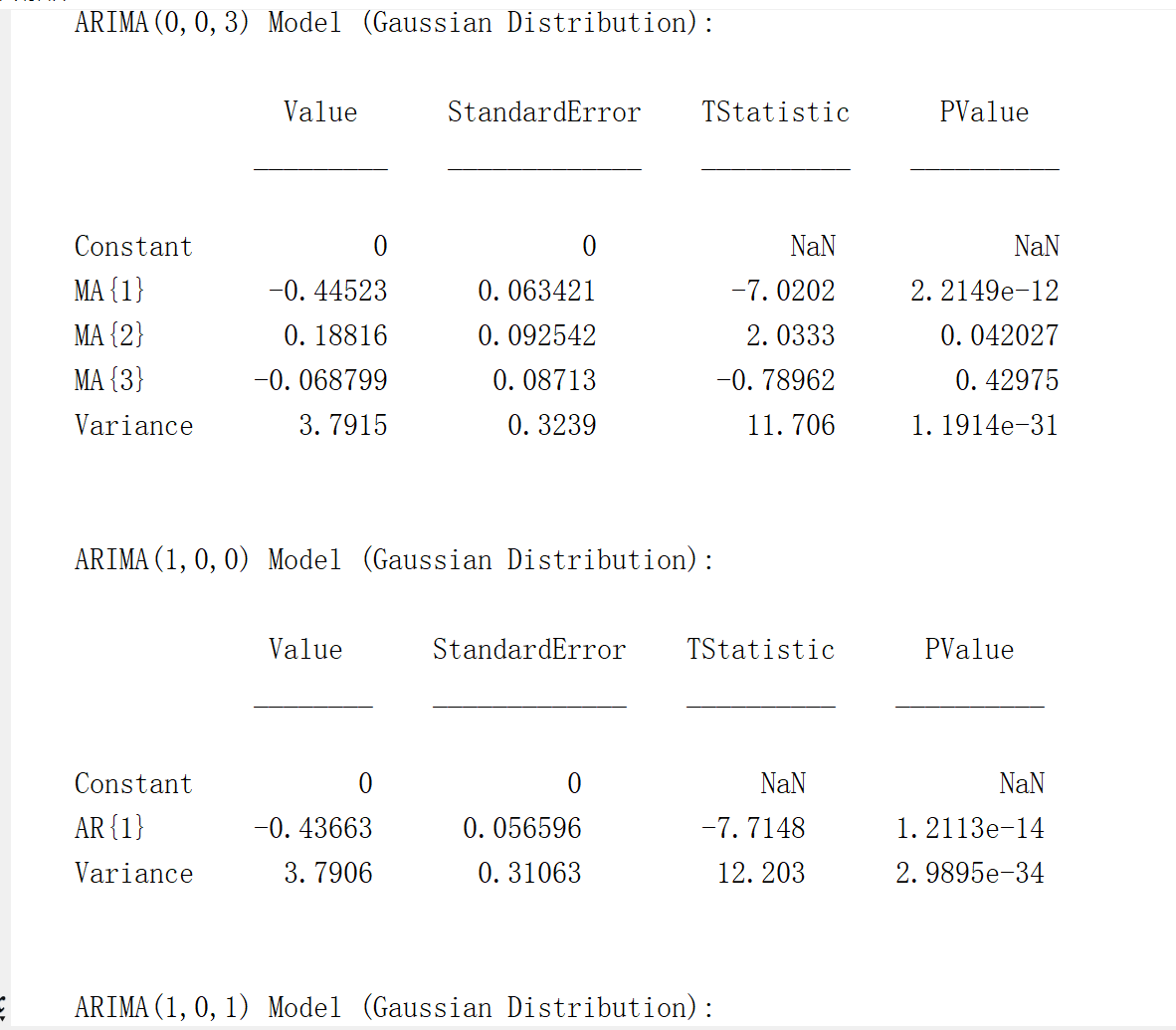4.定阶

fprintf('R,M,AIC,BIC的对应值如下\n%f'); % 根据AIC、BIC准则定阶
check = [R',M',aic',bic']

在命令行窗口显示R，M，AIC，BIC的值，以列向量的形式表示，然后根据AIC，BIC准则来进行选择模型的阶数。通常，取AIC，BIC最小的值，当AIC与BIC取值冲突时，以AIC为准。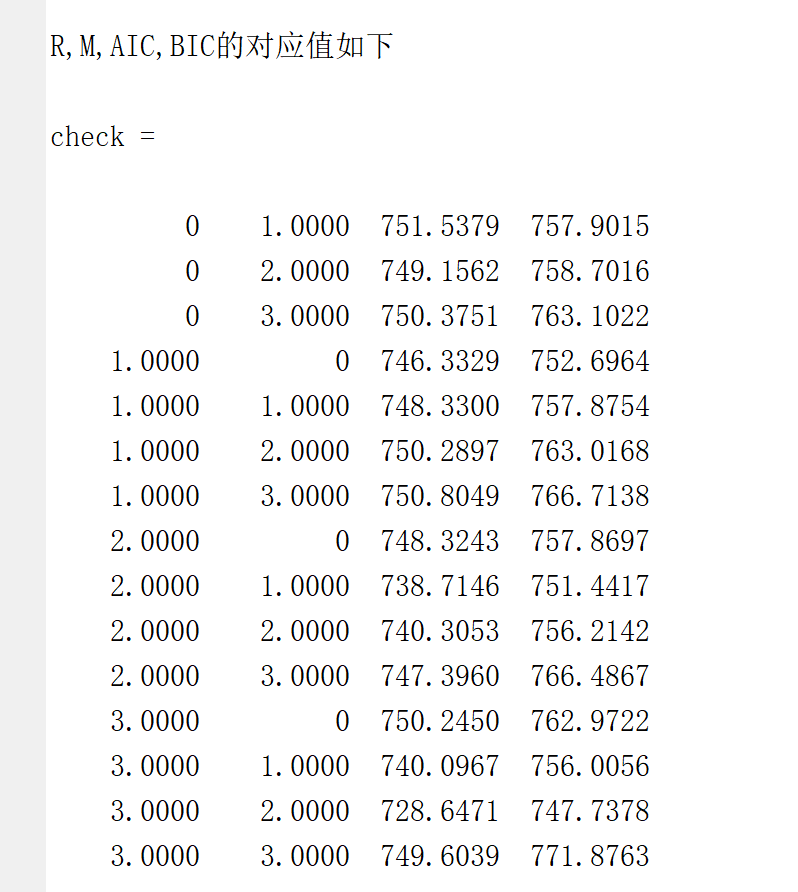例如第一个751.5379代表的是(0,1)  第四个746.3329代表的是(1,0)

5.代入，出结果

r = input('输入阶数R = ');m = input('输入阶数M = ');
ToEstMd = arima('ARLags',1 : r,'MALags',1:m,'Constant',0); %指定模型的结构

%% estimate && forecast && results
[EstMd,EstParamCov,logL,info] = estimate(ToEstMd,w');%模型拟合
w_Forecast = forecast(EstMd,n,'Y0',w');
yhat = y(end) + cumsum(w_Forecast); %一阶差分的还原值
for j = 1:n
x(m1 + j) = yhat(j) + x(m1+j-s); %x的预测值
end
xhat = x(m1 + 1 : end); % 提取x预测的值

分别输入r和m，然后进行计算，estimate函数进行模型拟合，然后forecast函数进行预测。之后进行一阶差分的还原，最后获得x的预测值。

Figure 6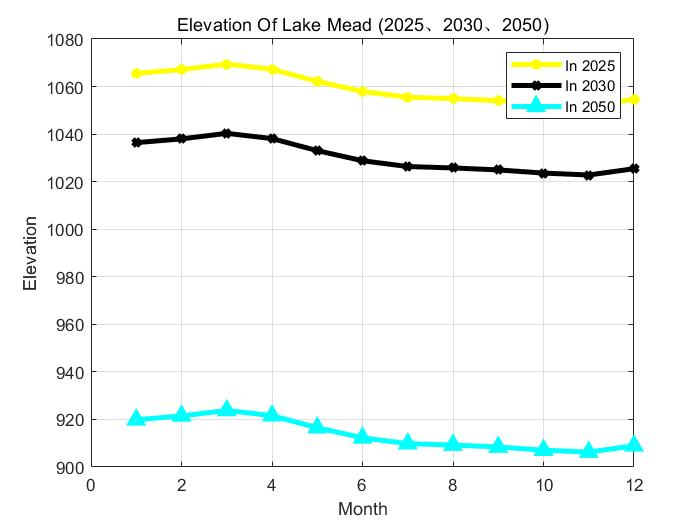这是预测结果的一些图像

画图的代码放下面了，有兴趣可以看看

%% result plot
clear
clc
d_25 = D(49 : 60);
d_30 = D(109 : 120);
d_50 = D(end - 11:end);

figure(6);
fst = plot(d_25);
fst.LineWidth = 3;
fst.Color = 'y';
fst.Marker = '*';
grid on;
hold on;

scd = plot(d_30);
scd.LineWidth = 3;
scd.Color = 'k';
scd.Marker = 'x';

hold on;
thd = plot(d_50);
thd.LineWidth = 3;
thd.Color = 'c';
thd.Marker = '^';

legend('In 2025','In 2030', ...
'In 2050');

xlabel('Month');
ylabel('Elevation');
title('Elevation Of Lake Mead (2025、2030、2050)');

鉴于本人水平有限，有错误的地方还恳请大家指出，批评，共同进步。

展开全文开发语言 机器学习 算法
• 一段程序，关于ARIMA模型的预测程序，求高人指点高人指点，结果图7不知道怎么发不上去，我是在R2010a上运行的，希望高手们指出问题所在。y=[ 7.94 7.38 7.34 8 7.43 7.04 7.64 8.55 8.39 8.24 7.25 6.79 6.71 6.09 ...

一段程序，关于ARIMA模型的预测程序，求高人指点高人指点，结果图7不知道怎么发不上去，我是在R2010a上运行的，希望高手们指出问题所在。

y=[   7.94    7.38    7.34    8    7.43    7.04    7.64    8.55    8.39    8.24    7.25    6.79    6.71    6.09    5.42    5.31    5.19    5.28    5.77    5.96    6.65    6.89    7.24    6.9    6.25    5.25    5.34    5.28    4.9    5.05    5.27    4.67    4.25    4.88    4.38    4.24    4.35    5.05    5.49    5.56    4.86    5.83    6.15    6.07    5.93    7.1    7.9    7.13    6.19    6.13    5.82    5.64    6.62    6.79    5.39    7.03    7.71    6.15    8.05    7.96    7.48    8.1    8.92    10.04    8.16    7.57    6.41    6.79    6.63    6.21    5.06    4.6    4.73    6.89    5.47    5.02    4.74    4.94    5.67    6.53    5.55    4.83    4    3.25    2.8    2.2    2.93    2.43    2.71    3.2    3.28    0    0    0    0    0    0    0    0    0    0    0    0    0    0    0    0    0    0    0    0    0    0    0    0    0    0    0    0    0    0    0    4.53    5.75    5.06    5.22    4.94    4.34    3.92    3.9    3.89    3.66    3.76    4.29    4.74    5.09    5.53    5.62    5.99    6.24    5.92    5.83    6.82    6.46    6.21    6.68    7.43    6.9    7.06    6.95    7.09    7.57    6.79    8.14    7.91    7.05    7.12    7.75    7.57    7.04    7.04    6.97    6.5    5.91    5.32    6.25    6.54    6.8    6.86    7.62    8.32    8.4    9.3    9.42    9.15    8.7    8.1    8.61    7.53    7.74    7.2    7.75    7.62    7.15    6.7    6.18    6.64    7.13    7.82    8.03    7.1    6.48    7.01    6.45    5.51    5.4    5.35    5.33    4.96    4.84 ];

ls=length(y);

figure(1);

plot(y);

set(gca,'Xlim',[0 ls]);

figure(2);

subplot(2,1,1);

set(gca,'Xlim',[0 ls]);

autocorr(y);

subplot(2,1,2)

parcorr(y);

DX=y;

for i=1:10;

if H==1

break;

else

DX=diff(y,i);

end

end

figure(3);

plot(DX);

set(gca,'Xlim',[0 ls]);

figure(4);

subplot(2,1,1)

autocorr(DX);

subplot(2,1,2)

parcorr(DX);

set(gca,'Xlim',[0 ls]);

%下面要对差分以后的序列进行拟合和预测，求出最好的阶数

z=[DX;zeros(12,1)];

z=iddata(z);

test=[];

for p=1:12

for q=1:12

m=armax(z(1:200),[p q]);

AIC=aic(m);

test=[test;p q AIC];

end

end

for k=1:size(test,1)

if test(k,3)==min(test(:,3))

p_test=test(k,1);

q_test=test(k,2);

break;

end

end

%拟合

m1=armax(z(1:200),[p_test q_test]);

figure(5);

e = resid(m1,z);

plot(e);

set(gca,'Xlim',[0 ls]);

figure(6);

subplot(2,1,1)

autocorr(e.outputdata)

subplot(2,1,2)

parcorr(e.outputdata)

set(gca,'Xlim',[0 ls]);

%预测过程

pr=predict(m1,z,12);

po=pr.outputdata;

figure(7)

plot(po,'r')

hold on

plot(y,'b');

set(gca,'Xlim',[0 ls]);

hold off

展开全文• arima的pq值matlab代码股票分析 用于简化股票技术分析要素的软件包。 该软件包旨在成为您开发自己的软件包的起点。 因此，所有安装/设置说明都假设您将继续开发。 设置 # should install requirements.txt packages ...
• ## ARIMA模型

万次阅读 多人点赞 2018-02-02 09:25:38
ARIMA模型 简介 ARIMA模型是由AR、I与MA模型组成 AR(p)：auto regressive，自回归模型，表示当前的数值与过去p个时间节点的值的回归，不依赖别的值，所以称为自回归；其中p" role="presentation">ppp称为自回归的...

## ARIMA模型

### 简介

• ARIMA模型是由AR、I与MA模型组成
• AR(p)：auto regressive，自回归模型，表示当前的数值与过去p个时间节点的值的回归，不依赖别的值，所以称为自回归；其中 p p $p$称为自回归的阶数。
• I(d)：integrateed，有的时间序列不是平稳信号，使用对数或者差分的方法可是将数据转化为平稳数据，数据的平稳性可以用数据的ACF(自相关)与PACF(偏自相关)图去判断。$d$$d$是差分的阶数
• MA(q)：moving average，移动平均模型，表示当前的值，是过去q个时间点的预测误差的回归。 q q <script type="math/tex" id="MathJax-Element-189">q</script>是MA的移动平均的阶数
• 具体的公式参考链接：http://danzhuibing.github.io/ml_arima_basic.html
• 关于ACF与PACF的解释：http://www.cnblogs.com/tongji-wu/p/3439372.html

### 代码

%%
clc,clear,close all

t = 1:100;
t = t';
y = 2*t + 10*sin(t/2) + randn( size(t) );
figure
plot( t, y )

%% ACF和PACF
figure
subplot(211),autocorr( y );
subplot(212),parcorr( y );
figure
dy = diff( y );
subplot(211),autocorr( dy );
subplot(212),parcorr( dy );

%% ARIMA 模型
Mdl = arima(5,1,0);
EstMdl = estimate(Mdl,y);
res = infer(EstMdl,y);
% 模型验证
figure
subplot(2,2,1)
plot(res./sqrt(EstMdl.Variance))
title('Standardized Residuals')
subplot(2,2,2),qqplot(res)
subplot(2,2,3),autocorr(res)
subplot(2,2,4),parcorr(res)
% 预测
[yF,yMSE] = forecast(EstMdl,20,'Y0',y);
UB = yF + 1.96*sqrt(yMSE);
LB = yF - 1.96*sqrt(yMSE);

figure
h4 = plot(y,'b');
hold on
h5 = plot(101:120,yF,'r','LineWidth',2);
h6 = plot(101:120,UB,'k--','LineWidth',1.5);
plot(101:120,LB,'k--','LineWidth',1.5);
hold off

• 首先观察数据是否为平稳序列，如果不是，则需要使用差分等方法进行转化，才能使用ARMA模型
• 一些结果
• 原始图像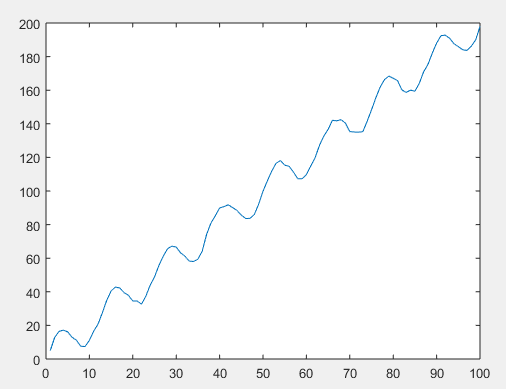• 原始数据的ACF与PACF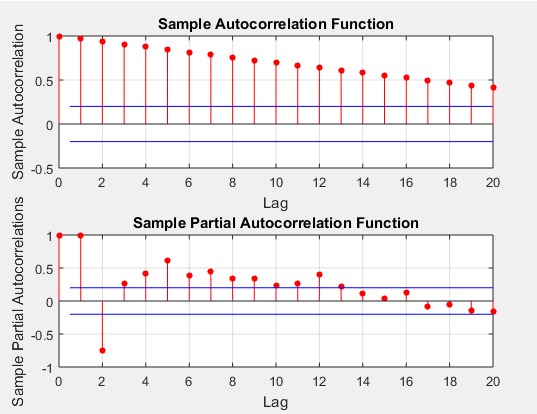• 原始数据差分后的ACF与PACF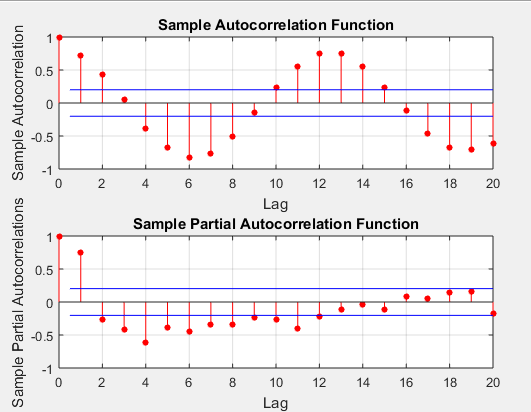• 模型验证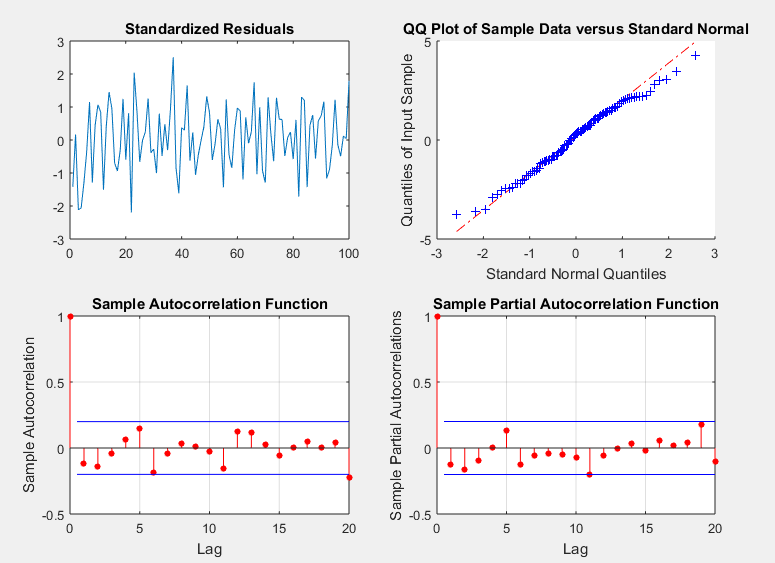• 模型预测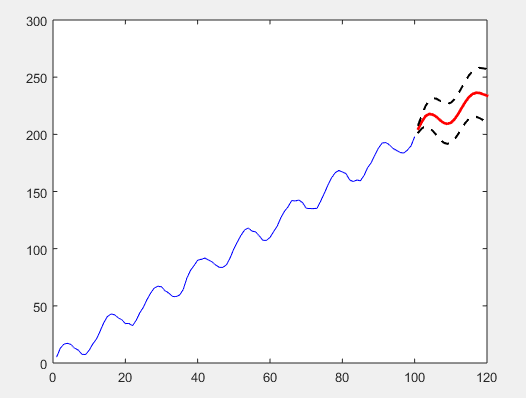展开全文时间序列
• arma 模型 matlab 代码 预测 简单实现预测的功能
• ar模型matlab代码纸2 该存储库包含使用以下时间序列预测的MATLAB代码：（i）ARIMA模型的MMSE预测（ii）卡尔曼滤波方法（iii）人工神经网络。 上面技术的小波版本的代码也显示在这里。 由于中心思想相同，因此降雨...
• ARMA时间序列模型matlab代码复杂系统的建模和分析 复杂系统建模与分析课程期末考试的报告和 MATLAB 代码，由 G. Nunnari 教授教授。 描述 本文旨在说明卡塔尼亚大学 Giuseppe Nunnari 教授在复杂系统建模和分析学术...
• arimamatlab代码时间序列预测 Python 简介 使用 AR、MA、ARMA、ARIMA、SARIMA 和具有预测评估的 Prophet 模型在 Python 中进行时间序列预处理和预测的介绍。
• arimamatlab代码time_series_forecasting_pytorch 实验源码：使用pytorch进行时间序列预测，包括MLP、RNN、LSTM、GRU、ARIMA、SVR、RF和TSR-RNN模型。 要求 Python 3.6.3（Python） keras 2.1.2 火炬 1.0.1 张量流...
• 自回归（AR）模型、移动平均（MA）模型、自回归移动平均（ARMA）和自回归差分移动平均（ARIMA模型是时间序列模型，它们主要是使用历史时间步的观测值作为回归方程的输入，以预测下一时间步的值。这是一个非常简单...
• 数学建模中的ARMA模型和ARIMA模型的使用实例（含代码） 对于较少时间段的时间预测，因为数据量较少，所以直接使用神经网络是不现实的，这里用的比较多的是时间序列模型预测和灰色预测，这里介绍一下时间序列中ARMA...数据
• arima的pq值matlab代码时间序列-预测 这是一个提供时间序列预测功能的 Java 开源库。 它是加法模型的实现。 该库由 Workday 的 Syman 团队发布，用于支持某些 Workday 产品中的基本时间序列预测功能。 如何使用 为了...
• arma模型matlab代码time_series_analysis 我在2020年S1和S2教授的统计课程“时间序列分析”的所有代码和软件包 我完全使用Python，R，Matlab / Octave，Julia和Stata（在C ++中用于R和Matlab的后端编程），除非某些...
• %% Forecast Conditional Mean and Variance Model % Step 1. Load the data and fit a model. load Data_EquityIdx nasdaq = DataTable....model = arima('ARLags',1,'Variance',garch(1,1),... 'Distribution',.
• 这是使用ARIMA模型预测股市价格的整体项目。 为了更好的可读性，下面列出了此存储库的内容： 1）MATLAB_Code文件夹：此文件夹包含用于2011年至2020年的ARIMA预测的完整工作MATLAB代码以及SENSEX数据集。 2）Python_......

# arima模型matlab代码matlab 订阅